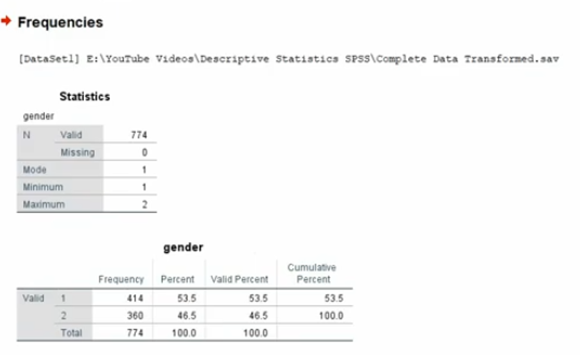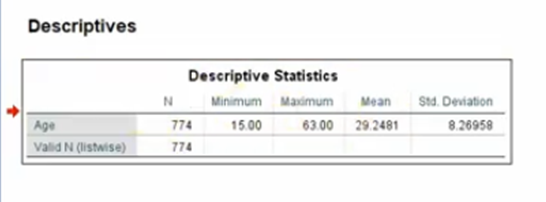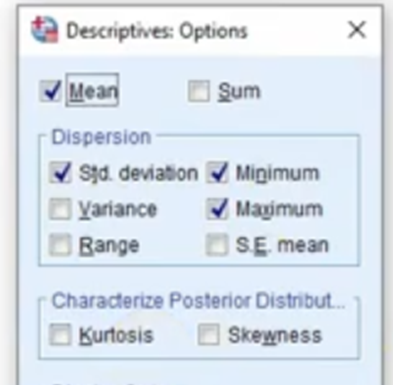# Descriptive Statistics using SPSS

Learn how to Run, Interpret, and Report Descriptive Statistics using SPSS

## Descriptive Statistics using SPSS

Once you know that data is free of obvious data entry errors, you can start the descriptive portion of your research (Learn More About Identification and Correction of Data Entry Errors). Mostly the descriptive statistics are used in the methodology section of the theses. Descriptive statistics are used to summarize and present this data in a meaningful manner so that the underlying information is easily understood. This chapter presents some of the tools for summarizing various kinds of data with the help of SPSS. Some basic terms and concepts have also been briefly explained.

Data Analysis and Results starts with the presentation of descriptive statistics. The video tutorial provides a detailed guide on how to run, interpret, and report descriptive statistics in a paper/thesis using SPSS.

### What is Descriptive Statistics?

Descriptive statistics are numerical and graphical methods used to summarize data and bring forth the underlying information. The numerical methods include measures of central tendency and measures of variability.

Descriptive statistics in SPSS can be accessed by clicking Analyze Menu → Descriptive Statistics. Detailed information can be obtained using Frequencies, Descriptives, Explore or Crosstabs. There are, however, different procedures depending on whether you have a categorical or continuous variable. Some of the statistics (e.g. mean, standard deviation) are not appropriate if you have a categorical variable.

### Descriptive Statistics for Categorical Variables

To obtain descriptive statistics for categorical variables, you should use Frequencies. This will tell you how many people gave each response (e.g. Count of Male/Female respondents, How many Field Staff or How many respondents from Office Staff). It doesn’t make any sense asking for means, standard deviations etc. for categorical variables, such as sex or marital status.

#### Steps involved in obtaining descriptive statistics for categorical variables

1. Choose Analyze Descriptive StatisticsFrequencies.
2. Select variables of your interest from the left list box, and press the arrow button to drop it in the Variable(s) list box. More than one variable can be selected from the list of variable in the study using Shift key. In this case we select Gender, Job Rank and Occupation.
3. Click on OK.

The results are displayed in the output window. A total of 4 tables are displayed. First table Statistics summarizes the categorical variables under study. The table shows how much of the observations are valid and if there are any missing values in the data. N refers to the total number of observations.

Next set of table include Frequency table for Gender, Rank and Occupation#### Interpretation and reporting of Categorical Variables

From the output shown above, we know that there are 414 males (1) (53.5%) and 360 females (2) (46.5%) in the sample, giving a total of 774 respondents. Researchers must note the number of respondents in different subgroups of the sample. For some analyses (e.g. ANOVA), it is easier to have roughly equal group sizes. If you have very unequal group sizes, particularly if the group sizes are small, it may be inappropriate to run the analyses.

As mentioned above descriptive statistics are normally reported in the methodology section under the head of frequencies where each of the categorical variables can be reported separately.

### Descriptive Statistics Continuous Variables

For continuous variables (e.g. age) it is easier to use Descriptives, which will provide you with ‘summary’ statistics such as mean, median and standard deviation. Since you don’t want to count every single value if incase there are hundreds of values. Continuous variables can also be categorized and then it’s meaningful to take frequency distribution of the variable. This would be discussed in detail in the Transformation chapter. Variables on Scale (interval and Ratio) can be selected for descriptive statistics.

#### Steps involved in obtaining descriptive statistics for continuous variables

1. Choose Analyze Descriptive StatisticsDescriptives.
2. Select variables of your interest from the left list box, and press the arrow button to drop it in the Variable(s) list box. More than one variable can be selected from the list of variable in the study using Shift key. In this case we select Age and TNA1.
3. Click on OK.#### Interpretation and reporting of Continuous Variables

From the output shown above, we know that the average age of respondents in the study is 29.24 with standard deviation of 8.26. These results can be reported when you are discussing the sample for your study in the methods section.

Descriptive statistics in SPSS can also provide different statistics; one is the distribution of score on continuous variables (Skewness and Kurtosis). These statistics are important when using parametric statistical techniques (t-tests, ANOVA, Correlation or regression).

Skewness provides indication if the distribution is symmetric or not, while Kurtosis on the other hand provides information about the ‘peakedness’ of the distribution. If the distribution is perfectly normal, you would obtain a Skewness and kurtosis value of 0 (rather an uncommon occurrence in the social sciences).

Positive Skewness values indicate positive skew (scores clustered to the left at the low values). Negative Skewness values indicate a clustering of scores at the high end (right-hand side of a graph). Most researchers consider data to be approximately normal in shape if the Skewness and kurtosis values turn out to be anywhere from – 1.0 to + 1.0. In order to Display Skewness and Kurtosis on the output, Select Options Button after entering the variables in the Variable(s) list box, and select you will be shown the following dialog boxCheck Kurtosis and Skewness, Press Continue and then press OK. Now the Descriptive Table will show Skewness and Kurtosis.